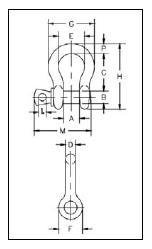# 1" ANCHOR SHACKLE

### Product Description :

#### WITH SCREW PINA = 42.9 mm = 1.69”

B = 28.7 mm = 1.23”

C = 95.5 mm = 3.76”

D = 25.4 mm = 1”

E = 68.5 mm = 2.70”

F = 60.5 mm = 2.38”

G = 119 mm = 4.69”

H = 167 mm = 6.57”

L = 14.2 mm = 0.56”

M = 129 mm = 5.68”

P = 31.8 mm = 1.25”